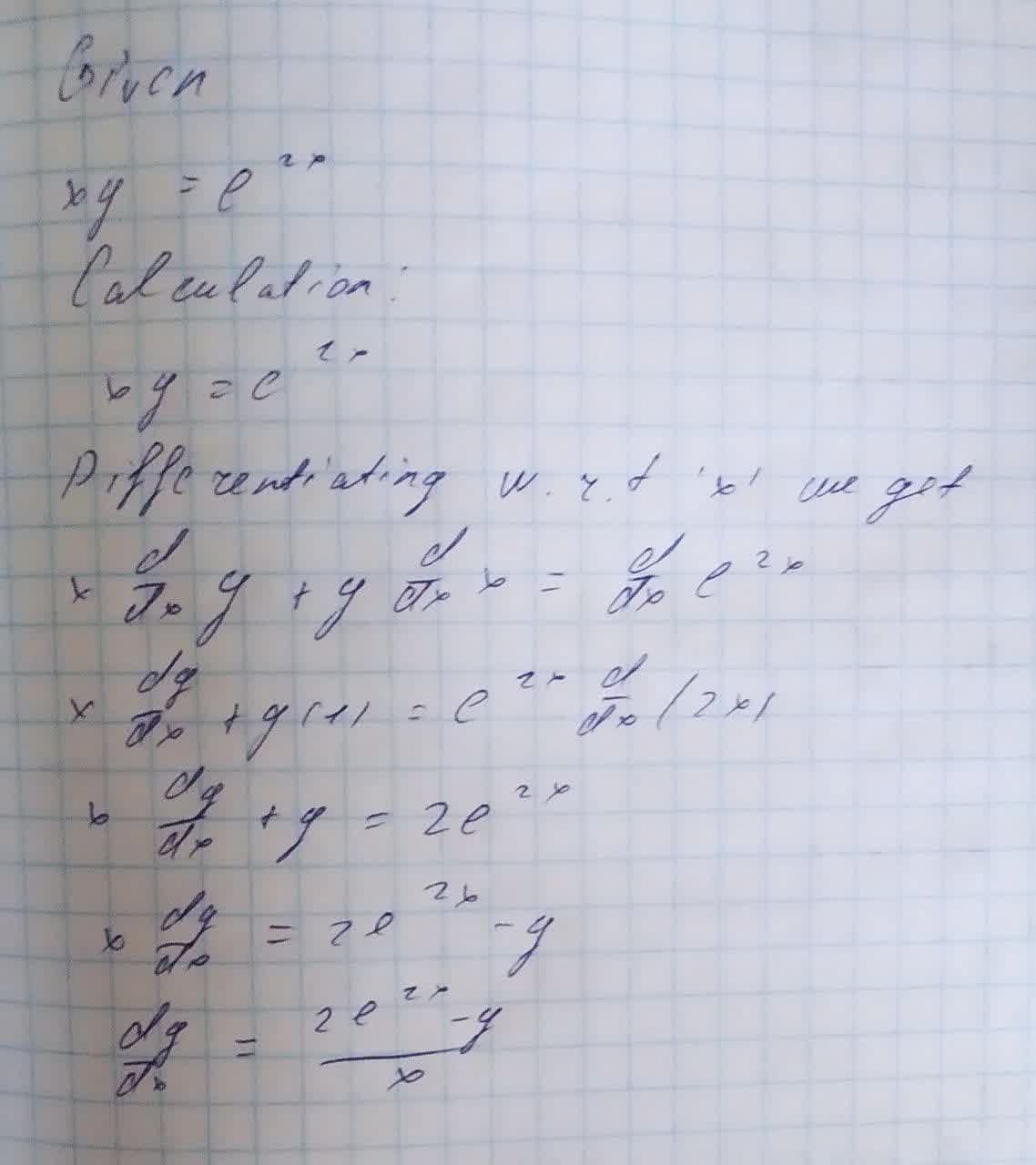Question# Find the indicative derivative of the following: xy=e^{2x}.y'

Derivatives
ANSWEREDFind the indicative derivative of the following:
$$\displaystyle{x}{y}={e}^{{{2}{x}}}.{y}'$$